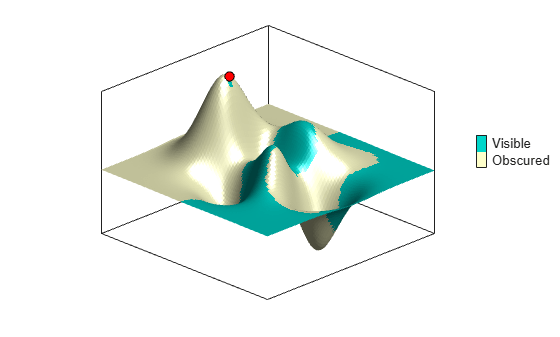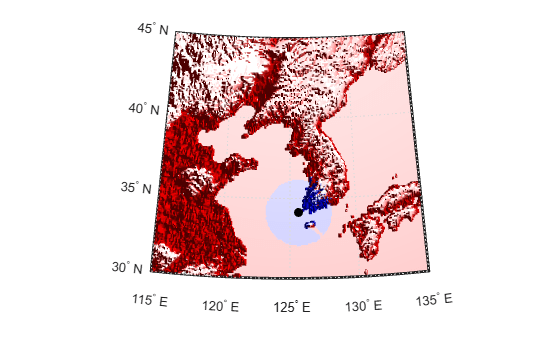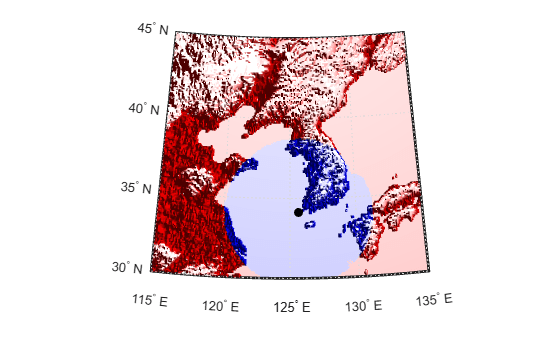# viewshed

Areas visible from point on terrain elevation grid

## Syntax

```[vis,R] = viewshed(Z,R,lat1,lon1) viewshed(Z,R,lat1,lon1,observerAltitude) viewshed(Z,R,lat1,lon1,observerAltitude,targetAltitude) viewshed(Z,R,lat1,lon1,observerAltitude,targetAltitude, ...   observerAltitudeOption) viewshed(Z,R,lat1,lon1,observerAltitude,targetAltitude, ...   observerAltidueOption,targetAltitudeOption) viewshed(Z,R,lat1,lon1,observerAltitude,targetAltitude, ...   observerAltitudeOption,targetAltitudeOption,actualRadius) viewshed(Z,R,lat1,lon1,observerAltitude,targetAltitude, ...   observerAltitudeOption,targetAltitudeOption, ...   actualRadius,effectiveRadius) ```

## Description

`[vis,R] = viewshed(Z,R,lat1,lon1)` computes areas visible from a point on a digital elevation grid. `Z` is a regular data grid containing elevations in units of meters. The observer location is provided as scalar latitude and longitude in units of degrees. The visibility grid `vis` contains `1`s at the surface locations visible from the observer location, and `0`s where the line of sight is obscured by terrain. `R` can be a geographic raster reference object, a referencing vector, or a referencing matrix.

If `R` is a geographic raster reference object, its `RasterSize` property must be consistent with `size(Z)`.

If `R` is a referencing vector, it must be a 1-by-3 with elements:

`[cells/degree northern_latitude_limit western_longitude_limit]`

If `R` is a referencing matrix, it must be 3-by-2 and transform raster row and column indices to or from geographic coordinates according to:

`[lon lat] = [row col 1] * R`

If `R` is a referencing matrix, it must define a (non-rotational, non-skewed) relationship in which each column of the data grid falls along a meridian and each row falls along a parallel. Nearest-neighbor interpolation is used by default. NaN is returned for points outside the grid limits or for which `lat` or `lon` contain NaN. All angles are in units of degrees.

`viewshed(Z,R,lat1,lon1,observerAltitude)` places the observer at the specified altitude in meters above the surface. This is equivalent to putting the observer on a tower. If omitted, the observer is assumed to be on the surface.

`viewshed(Z,R,lat1,lon1,observerAltitude,targetAltitude)` checks for visibility of target points a specified distance above the terrain. This is equivalent to putting the target points on towers that do not obstruct the view. If omitted, the target points are assumed to be on the surface.

```viewshed(Z,R,lat1,lon1,observerAltitude,targetAltitude, ...   observerAltitudeOption)``` controls whether the observer is at a relative or absolute altitude. If the `observerAltitudeOption` is `'AGL'`, then `observerAltitude` is in meters above ground level. If `observerAltitudeOption` is `'MSL'`, `observerAltitude` is interpreted as altitude above zero, or mean sea level. If omitted, `'AGL'` is assumed.

```viewshed(Z,R,lat1,lon1,observerAltitude,targetAltitude, ...   observerAltidueOption,targetAltitudeOption)``` controls whether the target points are at a relative or absolute altitude. If the target altitude option is `'AGL'`, the `targetAltitude` is in meters above ground level. If `targetAltitudeOption` is `'MSL'`, `targetAltitude` is interpreted as altitude above zero, or mean sea level. If omitted, `'AGL'` is assumed.

```viewshed(Z,R,lat1,lon1,observerAltitude,targetAltitude, ...   observerAltitudeOption,targetAltitudeOption,actualRadius)``` does the visibility calculation on a sphere with the specified radius. If omitted, the radius of the earth in meters is assumed. The altitudes, the elevations, and the radius should be in the same units. This calling form is most useful for computations on bodies other than the Earth.

```viewshed(Z,R,lat1,lon1,observerAltitude,targetAltitude, ...   observerAltitudeOption,targetAltitudeOption, ...   actualRadius,effectiveRadius)``` assumes a larger radius for propagation of the line of sight. This can account for the curvature of the signal path due to refraction in the atmosphere. For example, radio propagation in the atmosphere is commonly treated as straight line propagation on a sphere with 4/3 the radius of the Earth. In that case the last two arguments would be `R_e` and `4/3*R_e`, where `R_e` is the radius of the earth. Use `Inf` for flat Earth `viewshed` calculations. The altitudes, the elevations, and the radii should be in the same units.

## Examples

collapse all

Compute visibility for a point on the peaks map. Add the detailed information for the line of sight calculation between two points from `los2` .

Create the peak map and plot the visibility.

```Z = 500*peaks(100); refvec = [1000 0 0]; [lat1,lon1,lat2,lon2] = deal(-0.027,0.05,-0.093,0.042); [visgrid,visleg] = viewshed(Z,refvec,lat1,lon1,100); [vis,visprofile,dist,zi,lattrk,lontrk] ... = los2(Z,refvec,lat1,lon1,lat2,lon2,100); axesm('globe','geoid',earthRadius('meters')) meshm(visgrid,visleg,size(Z),Z); axis tight camposm(-10,-10,1e6) camupm(0,0) colormap(flipud(summer(2))) brighten(0.75) shading interp camlight h = lcolorbar({'obscured','visible'}); h.Position = [.875 .45 .02 .1]; plot3m(lattrk([1;end]),lontrk([1; end]), ... zi([1; end])+[100; 0],'r','linewidth',2) plotm(lattrk(~visprofile),lontrk(~visprofile), ... zi(~visprofile),'r.','markersize',10) plotm(lattrk(visprofile),lontrk(visprofile), ... zi(visprofile),'g.','markersize',10)```Compute the surface area visible by radar from an aircraft flying 3000 meters above the Yellow Sea. Assume that radio wave propagation in the atmosphere can be modeled as straight lines on a 4/3 radius Earth. This example also calculates the area visible to the plane above it at 5000 meters.

Load elevation data and a geographic cells reference object for the Korean peninsula, calculate the viewshed, and display it.

```load korea5c korea5c(korea5c<0) = -1; figure worldmap(korea5c,korea5cR) setm(gca,'geoid',[1 0]) da = daspect; pba = pbaspect; da(3) = 7.5*pba(3)/da(3); daspect(da); demcmap(korea5c) camlight(90,5); camlight(0,5); lighting gouraud material([0.25 0.8 0]) lat = 34.0931; lon = 125.6578; altobs = 3000; alttarg = 0; plotm(lat,lon,'wo') Re = earthRadius('meters'); [vmap,vmapl] = viewshed( ... korea5c,korea5cR,lat,lon,altobs,alttarg, ... 'MSL','AGL',Re,4/3*Re); meshm(vmap,vmapl,size(korea5c),korea5c)```Display the visible areas as blue and the obscured areas as red and drape the visibility colors on an elevation map, using lighting to bring out the surface topography. The aircraft's radar can see out a certain radius on the surface of the ocean, but note how some ocean areas are shadowed by the island of Jeju-Do. Also some mountain valleys closer than the ocean horizon are obscured, while some mountain tops further away are visible.

```caxis auto; colormap([1 0 0; 0 0 1]) lighting gouraud; axis off```Now calculate the area that the radar plane flying at an altitude of 3000 meters can have line-of-sight to other aircraft flying above it at 5000 meters. Note how the area is much larger but that some edges of the area are reduced by shadowing from Jeju-Do and the mountains on the Korean peninsula.

```[vmap,vmapl] = viewshed(korea5c,korea5cR,lat,lon,3000,5000, ... 'MSL','MSL',Re,4/3*Re); clmo surface meshm(vmap,vmapl,size(korea5c),korea5c) lighting gouraud```## Tips

The observer should be located within the latitude-longitude limits of the elevation grid. If the observer is located outside the grid, there is insufficient information to calculate a viewshed. In this case `viewshed` issues a warning and sets all elements of `vis` to zero.

## Version History

Introduced before R2006a